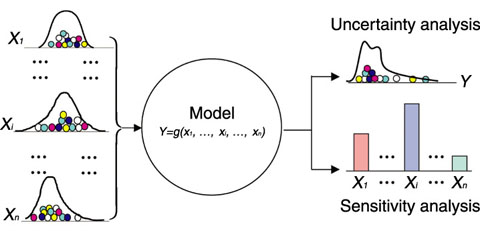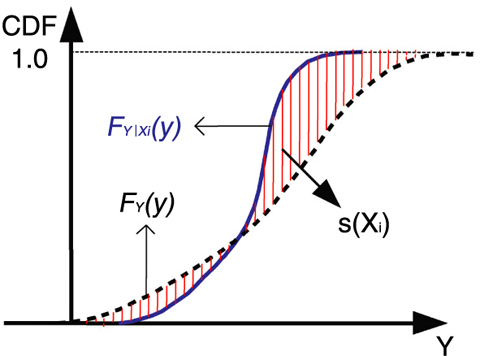# 6-6 Which Model Inputs Are More Important?

## －Development of a New Importance Measure and the GSALab Code－This Picture(71KB)

Fig.6-13　Concept of uncertainty and sensitivity analysis

Uncertainties in the model inputs {X1, …, Xi,…, Xn}(n is the number of model inputs) propagate through the model and result in uncertainty in the model output Y (the uncertainty of a model input or a model output is characterized by a probability distribution). While uncertainty analysis refers to the determination of the uncertainty in Y, sensitivity analysis refers to the determination of the contributions of the individual inputs to the uncertainty of Y.Fig.6-14　Basic idea of the new importance measure

FY(y) represents the cumulative distribution function (CDF) of the model output Y obtained with all model inputs {X1, …, Xi,…, Xn} being sampled randomly in their uncertainty ranges. FY|Xi(y) represents the conditional CDF of Y obtained with the model input of interest Xi being fixed and all other inputs being sampled randomly. The difference between FY(y) and FY|Xi(y) is evaluated by s(Xi), the area closed by these two CDFs.

Probabilistic Safety Assessment (PSA) is a systematic methodology to quantitatively evaluate the probabilities of occurrences of accidents and their consequences.

Given a PSA model of a nuclear power plant, there are uncertainties in the model inputs (e.g., the failure rates of components), which are usually characterized by probability distributions. Generally, Monte Carlo simulations are adopted to propagate input uncertainties through the PSA model and to obtain the probability distribution of the model output as well as its statistics, such as the variance and the mean. This process is called uncertainty analysis (UA) (Fig.6-13).

To reduce the uncertainty of Y, it is necessary to identify the model inputs that highly affect it. This is done through sensitivity analysis (SA) (Fig.6-13). The importance measure mostly used for SA is to find contributors from the model inputs to the variance of Y. If the variance of Y is greatly reduced due to reducing the variance of an input of interest, this input will be an important contributor to the variance of Y. In this method, it is implicitly assumed that the variance of Y is sufficient to describe the uncertainty of Y. However, if the distribution of Y is highly skewed, its variance will not be a good statistic to represent its uncertainty.

In our work we proposed a new importance measure, in which the quantified shape change of the CDF of Y is adopted as an indicator to evaluate the importance of each model input. As shown in Fig. 6-14, the area s(Xi) is used to evaluate the difference between FY(y) and FY|Xi(y). It is obvious that FY|Xi(y) is dependent on Xi, and so is s(Xi). Because there is uncertainty in Xi, each time we change the value of Xi in its uncertainty range and propagate the uncertainties of other model inputs through the model, we will obtain a new FY|Xi(y) as well as a new s(Xi). Repeating the above procedures thousands of times, we can obtain thousands of s(Xi). The expected value of s(Xi) can then be computed. We define this expected value as the importance measure of Xi.

This new importance measure was implemented in a computer code GSALab (Global Sensitivity Analysis Lab). In addition to PSA models, GSALab can also be applied to UA and SA of other models, such as atmospheric dispersion models and thermal-hydraulic models.

| | | | |